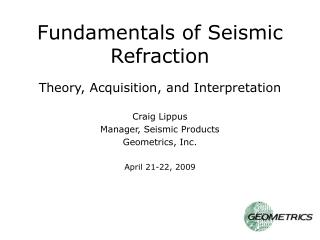DownloadDownload PresentationFundamentals of Seismic Refraction Theory, Acquisition, and Interpretation

# Fundamentals of Seismic Refraction Theory, Acquisition, and Interpretation

Download Presentation## Fundamentals of Seismic Refraction Theory, Acquisition, and Interpretation

- - - - - - - - - - - - - - - - - - - - - - - - - - - E N D - - - - - - - - - - - - - - - - - - - - - - - - - - -
##### Presentation Transcript

1. Fundamentals of Seismic RefractionTheory, Acquisition, and Interpretation Craig Lippus Manager, Seismic Products Geometrics, Inc. April 21-22, 2009

2. Fundamentals of Seismic Waves • What is a seismic wave?

3. Fundamentals of Seismic Waves • Transfer of energy by way of particle motion. • What is a seismic wave? Different types of seismic waves are characterized by their particle motion.

4. Three different types of seismic waves • Compressional (“p”) wave • Shear (“s”) wave • Surface (Love and Raleigh) wave Only p and s waves (collectively referred to as “body waves”) are of interest in seismic refraction.

5. Compressional (“p”) Wave Identical to sound wave – particle motion is parallel to propagation direction. Animation courtesy Larry Braile, Purdue University

6. Shear (“s”) Wave Particle motion is perpendicular to propagation direction. Animation courtesy Larry Braile, Purdue University

7. Velocity of Seismic Waves Depends on density and elastic moduli where K = bulk modulus,  = shear modulus, and  = density.

8. Velocity of Seismic Waves Bulk modulus = resistance to compression = incompressibility Shear modulus = resistance to shear = rigidity The less compressible a material is, the greater its p-wave velocity, e.g., sound travels about four times faster in water than in air. The more resistant a material is to shear, the greater its shear wave velocity.

9. Q. What is the rigidity of water?

10. A. Water has no rigidity. Its shear strength is zero. Q. What is the rigidity of water?

11. Q. How well does water carry shear waves?

12. A. It doesn’t. Q. How well does water carry shear waves?

13. Fluids do not carry shear waves. This knowledge, combined with earthquake observations, is what lead to the discovery that the earth’s outer core is a liquid rather than a solid – “shear wave shadow”.

14. p-wave velocity vs. s-wave velocity p-wave velocity must always be greater than s-wave velocity. Why? K and  are always positive numbers, so Vp is always greater than Vs.

15. Velocity – density paradox Q. We know that in practice, velocity tends to be directly proportional to density. Yet density is in the denominator. How is that possible?

16. Velocity – density paradox Q. We know that in practice, velocity tends to be directly proportional to density. Yet density is in the denominator. How is that possible? A. Elastic moduli tend to increase with density also, and at a faster rate.

17. Velocity – density paradox Note: Elastic moduli are important parameters for understanding rock properties and how they will behave under various conditions. They help engineers assess suitability for founding dams, bridges, and other critical structures such as hospitals and schools. Measuring p- and s-wave velocities can help determine these properties indirectly and non-destructively.

18. Q. How do we use seismic waves to understand the subsurface?

19. Q. How do we use seismic waves to understand the subsurface? • We must first understand wave • behavior in layered media.

20. Q. What happens when a seismic wave encounters a velocity discontinuity? A. Some of the energy is reflected, some is refracted. We are only interested in refracted energy!!

21. Seismic Wavefront • Seismic Ray • Huygen’s Principle • Snell’s Law • Reciprocity Five important concepts

22. Q. What is a seismic wavefront?

23. Q. What is a seismic wavefront? A. Surface of constant phase, like ripples on a pond, but in three dimensions.

24. Q. What is a seismic wavefront?

25. The speed at which a wavefront travels is the seismic velocity of the material, and depends on the material’s elastic properties. In a homogeneous medium, a wavefront is spherical. Velocity inhomogeneities have a distorting effect.

26. Seismic wavefront

27. Q. What is a ray?

28. Q. What is a ray? A. Also referred to as a “wavefront normal”, a ray is an arrow drawn perpendicular to the wavefront, indicating the direction of travel at that point on the wavefront. There are an infinite number of rays on a wavefront.

29. Ray

30. Huygens' Principle Every point on a wavefront can be thought of as a new point source for waves generated in the direction the wave is traveling.

31. Q. What causes refraction?

32. Q. What causes refraction? A. Different portions of the wavefront reach the velocity boundary earlier than other portions, speeding up or slowing down on contact, causing distortion of the wavefront.

33. (1) Snell’s Law

34. r approaches 90o as i increases Snell’s Law

35. Critical Refraction At the Critical Angle of incidence ic, angle of refraction r = 90o Snell’s Law (2) (3)

36. Critical Refraction At Critical Angle of incidence ic, angle of refraction r = 90o Snell’s Law

37. Critical Refraction At Critical Angle of incidence ic, angle of refraction r = 90o Snell’s Law

38. Critical Refraction Seismic refraction makes use of critically-refracted, first-arrival energy only. The rest of the waveform is ignored. Snell’s Law

39. Principal of Reciprocity The travel-time of seismic energy between two points is independent of the direction traveled, i.e., interchanging the source and the geophone will not affect the seismic travel-time between the two.

40. Using Seismic Refraction to Map the Subsurface Critical Refraction Plays a Key Role

41. (4) Refracted arrivals from layer 2 T2i

42. (4) Refracted arrivals from layer 2 T2i

43. (4) Refracted arrivals from layer 2 T2i

44. (4) Refracted arrivals from layer 2 T2i